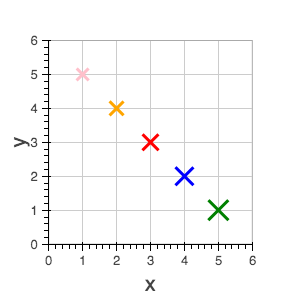# BokehJS Reference¶

## Data Sources¶

Data is typically passed to bokehjs through a `ColumnDataSource` which has named columns of data that are available to glyph renderers. Note that all the columns in a given `ColumnDataSource` should have the same length. It is possible and often necessary to have different data sources for different renderers.

Warning

JavaScript `null` values are not valid inputs to BokehJS renderers. Please be aware that they will be silently interpreted as zero.

## Line Properties¶

BokehJS exposes all the standard HTML5 canvas path stroking properties. All of these properties may take single values, or references to vectors of data. For instance, it is possible to draw multiple rectangles at once using the `rect` glyph. They may all be given the same `line_width` or each rectangle may be given its own `line_width`.

`line_color`
color to use to stroke lines with
`line_width`
line stroke width in units of pixels
`line_alpha`
floating point between 0 (transparent) and 1 (opaque)
`line_join`

how path segments should be joined together

`line_cap`

how path segments should be terminated

`line_dash`

a line style to use

• `'solid'`
• `'dashed'`
• `'dotted'`
• `'dotdash'`
• `'dashdot'`
• an array of integer pixel distances that describe the on-off pattern of dashing to use
• a string of spaced integers matching the regular expression ‘^(\d+(\s+\d+)*)?\$’ that describe the on-off pattern of dashing to use
`line_dash_offset`
the distance in pixels into the `line_dash` that the pattern should start from

## Fill Properties¶

BokehJS exposes all the standard HTML5 path filling properties. All of these properties may take single values, or references to vectors of data. For instance, it is possible to draw multiple rectangles at once using the `rect` glyph. They may all be given the same `fill_width`, or each rectangle may be given its own `fill_width`.

`fill_color`
color to use to fill paths with
`fill_alpha`
floating point between 0 (transparent) and 1 (opaque)

## Text Properties¶

BokehJS exposes most of the standard HTML5 text properties. All of these properties may take single values, or references to vectors of data. For instance, it is possible to draw multiple text items at once using the `text` glyph. They may all be given the same `font_size` or each line of text may be given its own `font_size`.

`text_font`
font name, e.g., `'times'`, `'helvetica'`
`text_font_size`
font size in px, em, or pt, e.g., `'12pt'`, `'1.5em'`
`text_font_style`

font style to use

• `'normal'` normal text
• `'italic'` italic text
• `'bold'` bold text
`text_color`
color to use to render text with
`text_alpha`
floating point between 0 (transparent) and 1 (opaque)
`text_align`
horizontal anchor point for text: `'left'`, `'right'`, `'center'`
`text_baseline`

vertical anchor point for text

• `'top'`
• `'middle'`
• `'bottom'`
• `'alphabetic'`
• `'hanging'`

Note

There is currently only support for filling text. An interface to stroke the outlines of text has not yet been exposed.

## Markers¶

Markers are a subset of BokehJS glyphs that have a uniform interface and are suitable for simple scatter type plots. All markers expose the following fields:

• `x`, `y` - center point coordinates
• `size` - screen units

and have Line Properties and Fill Properties as appropriate.

## Glyphs¶

Bokeh plots are centered around glyphs, which generally have some combination of line, fill, or text properties, depending on what is appropriate for a given glyph. For example, the `Circle` glyph has both line and fill properties, but the `Bezier` glyph only has line properties.

Note

Each of the glyph images below links to a live JSFiddle.

### `annular_wedge`¶

The annular_wedge glyph displays annular wedges centered at the given coordinates with the corresponding `start_radius`, `end_radius`, `start_angle` and `end_angle`.

Note

the `direction` field may be used to indicate which direction the drawing should occur between `start_radius` and `end_radius`.

• `x`, `y` - center point coordinates
• `start_radius`
• `end_radius`
• `start_angle`
• `end_angle`
• `direction`
• values: `'clock'`, `'anticlock'`
• default: `'anticlock'`
• Line Properties
• Fill Properties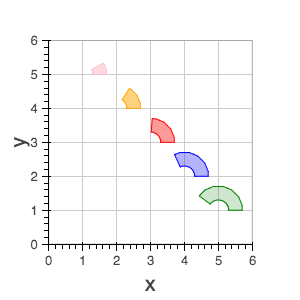### `annulus`¶

The annulus glyph displays annular rings centered at the given coordinates with the corresponding `start_radius` and `end_radius`.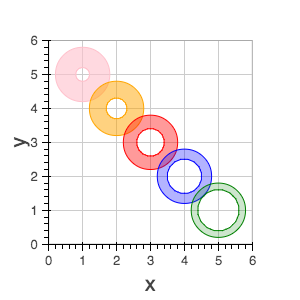### `arc`¶

The annulus glyph displays circular line arcs centered at the given coordinates with the corresponding `radius`, `start_angle` and `end_angle`.

Note

the `direction` field may be used to indicate which direction the drawing should occur between `start_radius` and `end_radius`.

• `x`, `y` - center point coordinates
• `radius`
• `start_angle`
• `end_angle`
• `direction`
• values: [`'clock'` or `'anticlock'`]
• default: `'anticlock'`
• Line Properties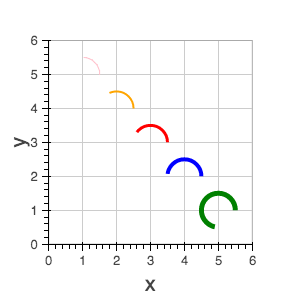### `asterisk`¶

The asterisk glyph is a marker that displays asterisks at the given coordinates.

• `x`, `y` - center point coordinates
• `size`
• Line Properties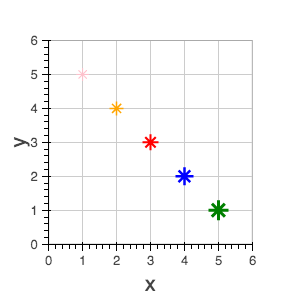### `bezier`¶

The bezier glyph displays Bezier curves with the given starting, ending, and control points.

• `x0`, `y0` - starting point coordinates
• `x1`, `y1` - ending point coordinates
• `cx0`, `cy0` - first control point coordinates
• `cx1`, `cy1` - second control point coordinates
• Line Properties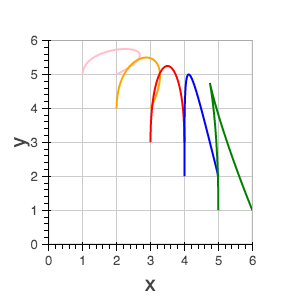### `circle`¶

The circle glyph has two forms, a marker form that takes a `size` field or a non-marker form that takes a `radius` field.

 `x`, `y` - center point coordinates `size` Line Properties Fill Properties `x`, `y` - center point coordinates `radius` Line Properties Fill Properties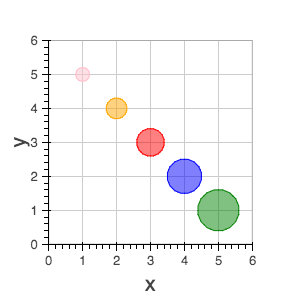### `circle_cross`¶

The circle_cross glyph is a marker that displays circles together with a crossbar (+) at the given coordinates.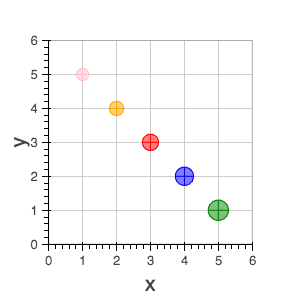### `circle_x`¶

The circle_x glyph is a marker that displays circles together with an X at the given coordinates.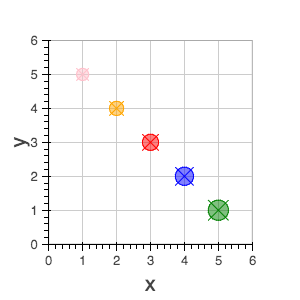### `cross`¶

The cross glyph is a marker that displays crossbar symbols (+) at the given coordinates.

• `x`, `y` - center point coordinates
• `size`
• Line Properties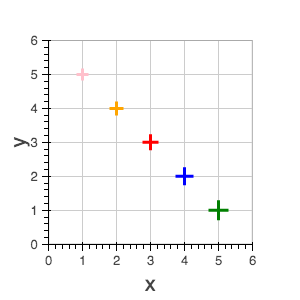### `diamond`¶

The diamond glyph is a marker that displays diamonds at the given coordinates.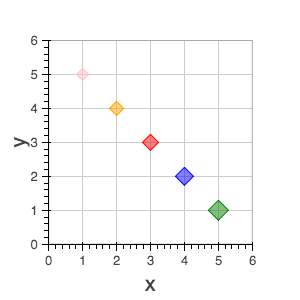### `diamond_cross`¶

The diamond_cross glyph is a marker that displays diamonds together with a crossbar (+) at the given coordinates.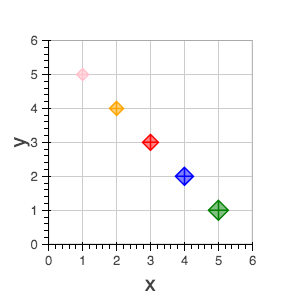### `image`¶

The image glyph has two forms. The first form takes each `image` as a one-dimensional array of scalar values together with `rows` and `cols` fields that describe the two-dimensional shape of the array. The second form takes each `image` as a”array of arrays” (assumed to be non-ragged) and the shape is inferred automatically. A `palette` (string name of a built-in palette, currently) must also be supplied to use for color-mapping the scalar image.

Note

The image glyph is vectorized like other glyphs, i.e. it may be used to display several images at once.

Warning

The second (2D) form is significantly less efficient. It is currently used by the python interface to send data to the browser, but may be deprecated in the future.

 `image` - 1D array of data `rows` - number of rows in image `cols` - number of columns in image `x`, `y` - lower left `dw` - width on screen `dh`- height on screen `palette` `image` - 2D array of data `x`, `y` - lower left `dw` - width on screen `dh`- height on screen `palette`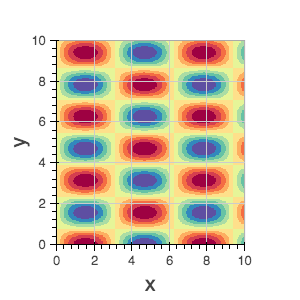### `image_rgba`¶

The image_rgba glyph has two forms. The first form takes each `image` as a one-dimensional array of RGBA values (encoded as 32-bit integers) together with `rows` and `cols` fields that describe the two-dimensional shape of the array. The second form takes each `image` as a “array of arrays” (assumed to be non-ragged) and the shape is inferred automatically.

Note

The image_rgba glyph is vectorized like other glyphs, i.e. it may be used to display several images at once.

Warning

The second (2D) form is significantly less efficient. It is currently used by the python interface to send data to the browser, but may be deprecated in the future.

 `image` - 1D array of RGBA `rows` - number of rows in image `cols` - number of columns in image `x`, `y` - lower left `dw` - width on screen `dh`- height on screen `image` - 2D array of RGBA `x`, `y` - lower left `dw` - width on screen `dh`- height on screen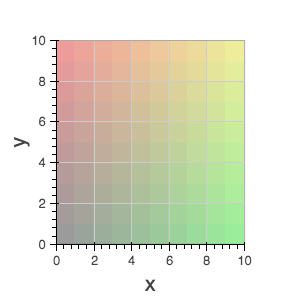### `image_url`¶

The image_url glyph accepts the URLs of an images to display. The images are centered on the given coordinates and rotated by the given angles.

• `x`, `y` - center point coordinates
• `url`
• `angle`

### `inverted_triangle`¶

The inverted_triangle glyph is a marker that displays upside-down triangles at the given coordinates.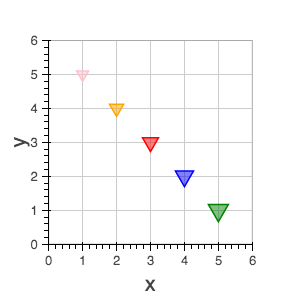### `line`¶

The line glyphs displays a single line that connects several points given by the arrays of coordinates `x` and `y`.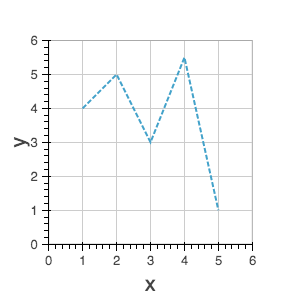### `multi_line`¶

The multi_line glyphs displays several lines, each with points given by the arrays of coordinates that are the elements of `xs` and `ys`. This glyph is especially useful for implementing parallel coordinates plots, or plotting several aligned series simultaneously.

Note

For this glyph, the vector data is not simply an array of scalars, it is really an “array of arrays”.

• `xs`, `ys` - lists of line coordinates
• Line Properties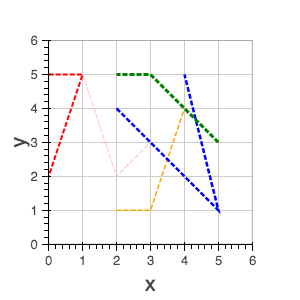### `oval`¶

The oval glyph displays ovals centered on the given coordinates with the given dimensions and angle.

• `x`, `y` - center point coordinates
• `width`
• `height`
• `angle`
• default: 0
• Line Properties
• Fill Properties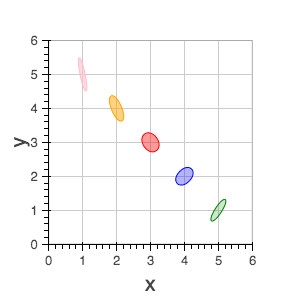### `patch`¶

The line glyphs displays a single polygonal patch that connects several points given by the arrays of coordinates `x` and `y`.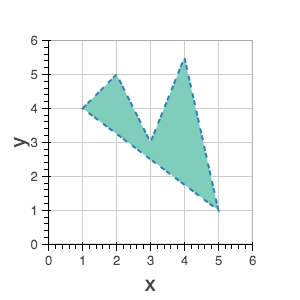### `patches`¶

The patches glyphs displays several patches, each with points given by the arrays of coordinates that are the elements of `xs` and `ys`. This glyph is especially useful for implementing stacked area charts and cartograms.

Note

For this glyph, the vector data is not simply an array of scalars, it is really an “array of arrays”.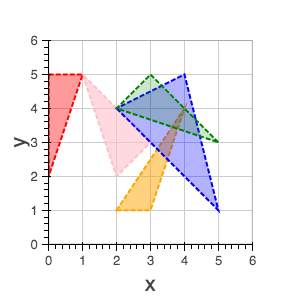### `quad`¶

The quad glyph displays axis-aligned rectangles with the given dimensions.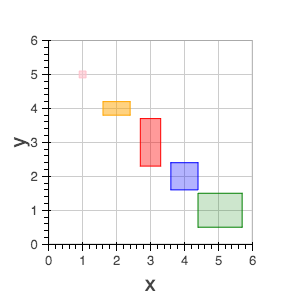### `quadratic`¶

The quadratic glyph displays quadratic curves with the given starting, ending, and control points.

• `x0`, `y0` - starting point coordinates
• `x1`, `y1` - ending point coordinates
• `cx`, `cy` - control point coordinates
• Line Properties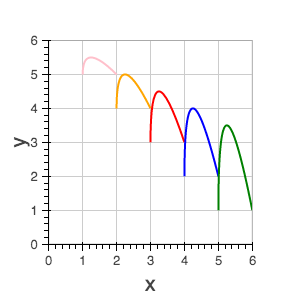### `ray`¶

The ray glyph displays line segments starting at the given coordinate and extending the given `length` at the given `angle`.

• `x0`, `y0` - starting point coordinates
• `length` - screen units
• `angle`
• default: 0
• Line Properties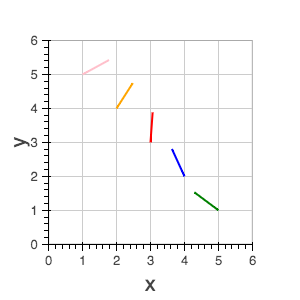### `rect`¶

The rect glyph displays rectangles centered on the given coordinates with the given dimensions and angle.

• `x`, `y` - center point coordinates
• `width`
• `height`
• `angle`
• default: 0
• Line Properties
• Fill Properties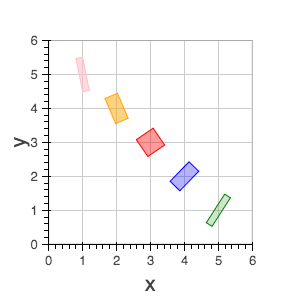### `segment`¶

The segment glyph displays line segments with the given starting and ending coordinates.

• `x0`, `y0` - starting point coordinates
• `x1`, `y1` - ending point coordinates
• Line Properties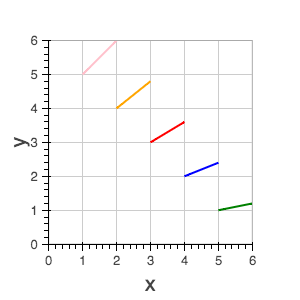### `square`¶

The square glyph is a marker that displays squares at the given coordinates.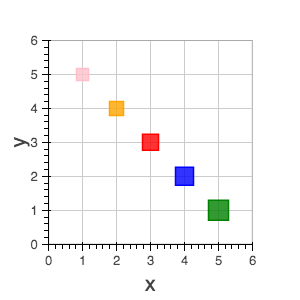### `square_cross`¶

The square_cross glyph is a marker that displays squares together with a crossbar (+) at the given coordinates.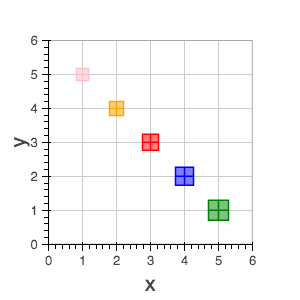### `square_x`¶

The square_x glyph is a marker that displays squares together with an X at the given coordinates.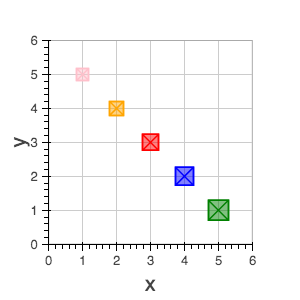### `text`¶

The text glyph displays text at the given coordinates rotated by the given angle. The location of the coordinates relative to the text is indicated by the text properties.

• `x`, `y` - text coordinates (positioning determined by text properties)
• `text`
• `angle`
• default: 0
• Text Properties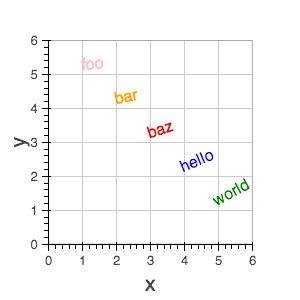### `triangle`¶

The triangle glyph is a marker that displays triangles at the given coordinates.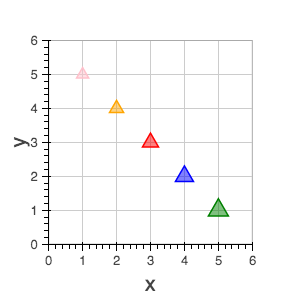### `wedge`¶

The annular_wedge glyph displays circular wedges centered at the given coordinates with the corresponding `radius`, `start_angle` and `end_angle`.

Note

the `direction` field may be used to indicate which direction the drawing should occur between `start_radius` and `end_radius`.

• `x`, `y` - center point coordinates
• `radius`
• `start_angle`
• `end_angle`
• `direction`
• values: [`'clock'` or `'anticlock'`]
• default: `'anticlock'`
• Line Properties
• Fill Properties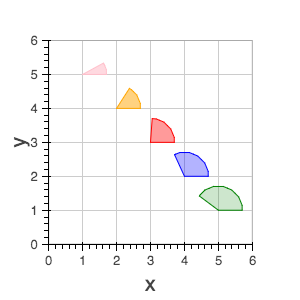### `x`¶

The x glyph is a marker that displays X symbols at the given coordinates.